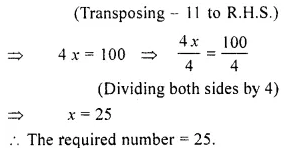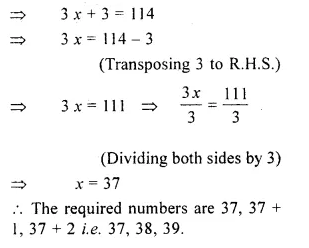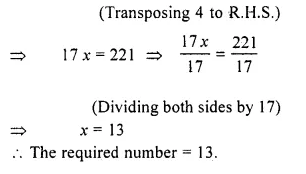## RS Aggarwal Class 6 Solutions Chapter 9 Linear Equations in One Variable Ex 9C

These Solutions are part of RS Aggarwal Solutions Class 6. Here we have given RS Aggarwal Solutions Class 6 Chapter 9 Linear Equations in One Variable Ex 9C.

Other Exercises

Question 1.
Solution:
Let the required number be x.
Then, x + 9 = 36
=> x = 36 – 9
(Transposing 9 to R.H.S.)
=> x = 27
The required number = 27.

Question 2.
Solution:
Let the required number be x. Then,
4x – 11 = 89
=> 4x = 89 + 11Question 3.
Solution:
Let the required number be x. Then,
x x 5 = x + 80
=> 5x = x + 80
=> 5x – x = 80Question 4.
Solution:
Let the three consecutive numbers be x,
x + 1 and x + 2. Then,
x + (x + 1) + (x + 2) = 114
=> x – x + 1 + x + 2 = 114Question 5.
Solution:
Let the required number be x. Then
x x 17 + 4 = 225
=> 17x + 4 = 225
=> 17x = 225 – 4Question 6.
Solution:
Let the required number be x. Then
3 x + 5 = 50
=> 3 x = 50 – 5Question 7.
Solution:
Let the smaller number be x.
Then the other number = x + 18
According to question,
x + (x + 18) = 92
=> 2 x + 18 = 92
=> 2 x = 92 – 18
(Transposing 18 to R.H.S.)
=> 2 x = 74
=> x = 37
(Dividing both sides by 2)
One number = 37
Another number = 37 + 18
= 55.

Question 8.
Solution:
Let the smaller number be x.
Then, the other number = 3 x
According to question, x + 3 x = 124
=> 4 x = 124Question 9.
Solution:
Let the smallest number be x.
Then, the other number = 5 x
According to question,
5 x – x = 132
=> 4 x = 132Question 10.
Solution:
Let the two consecutive even number be x and x + 2.
Then x + x + 2 = 74
=> 2 x + 2 = 74
=> 2 x = 74 – 2
(Transposing 2 to RHS.)
=> 2x = 72
=> x = 36
(Dividing both sides by 2)
The required numbers are 36 and (36 + 2) i.e. 36 and 38.

Question 11.
Solution:
Let the three consecutive odd numbers be x, (x + 2) and (x + 4).
According to the question,
x + (x + 2) + (x + 4) = 21
3x + 6 = 21
=> 3 x = 21 – 6
(Transposing 6 to R.H.S.)
=> 3 x = 15
=> x = 5
(Dividing both sides by 3)
The required numbers are 5, 5 + 2 and 5 + 4 i.e. 5, 7 and 9.

Question 12.
Solution:
Let the present age of Ajay be x years. Then, the present age of Reena = (x + 6) years
According to the question,
x + (x + 6) = 28
2x + 6 = 28
=> 2x = 28 – 6
(Transposing 6 to R.H.S.)
=> 2 x = 22
=> x = 11
(Dividing both sides by 2)
Present age of Ajay = 11 years
and present age of Reena = 11 + 6
= 17 years.

Question 13.
Solution:
Let the present age of Vikas be x years.
Then, the present age of Deepak = 2x years
According to the question,
2x – x = 11
=> x = 11
Present age of Vikas = 11 years
and present age of Deepak = 2 x 11
= 22 years.

Question 14.
Solution:
Let the present age of Rekha be x years
Then, the present age of Mrs. Goel = (x + 27) years
Rekha’s age after 8 years = (x + 8) years
Mrs. Goel’s age after 8 years = (x + 27 + 8) years
= (x + 35) years
According to the question,
x + 35 = 2 (x + 8)
=> x + 35 = 2x + 16
=> x – 2x = 16 – 35
(Transposing 2x to L.H.S. and 35 to R.H.S.)
=> – x = – 19
=> x = 19
Present age of Rekha =19 years and present age of Mr. Goel = 19 + 27 = 46 years.

Question 15.
Solution:
Let the present age of the son be A years.
Then, the present age of the man
= 4 x years
Son’s age after 16 years = (x + 16) years Man’s age after 16 years
= (4 x + 16) years According to the question,
4x + 16 = 2 (x + 16)
=> 4x + 16 = 2x + 32
=> 4x – 2x = 32 – 16
(Transposing 2 x to L.H.S. and 16 to R.H.S.)
=> 2x = 16
=> x = 8 (Dividing both sides by 2)
Present age of the son = 8 years and present age of the man = 8 x 4 = 32 years.

Question 16.
Solution:
Let the present age of the son be x years.
Then, the present age of the man = 3x years
five years ago, the age of the son = (x – 5) years
five years ago, the age of the man = (3x – 5) years
According to the question, 3x – 5 = 4(x – 5)
=>3x – 5 = 4x – 20
=>3x – 4x = – 20 + 5
(Transposing 4 x to L.H.S. and – 5 to R.H.S.)
=> – x = – 15 => x = 15
Present age of the son = 15 years and present age of the man = 3 x 15 = 45 years.

Question 17.
Solution:
Let the present age of Fatima be A years. According to the question,
x + 16 = 3 x
=> x – 3x = – 16
(Transposing 3 x to L.H.S. and 16 to R.H.S.)
=> – 2 x = – 16
=> x = 8
(Dividing both sides by – 2)
Present age of Fatima = 8 years.

Question 18.
Solution:
Let the present age of Rahim be x years
Rahim’s age after 32 years = (x + 32) years
Rahim’s age 8 years ago = (x – 8) years
According to the question, x + 32 = 5 (x – 8)
=> x + 32 = 5 x – 40
=> x – 5x = – 40 – 32
(Transposing 5 x to L.H.S. and 32 to R.H.S.)
– 4 x = – 72
x= 18
(Dividing both sides by – 4)
Present age of Rahim =18 years

Question 19.
Solution:
Let the number of 50 paisa coins be x.
Then, the number of 25 paisa coins = 4x
Total value of 50 paisa coins = 50 x paisa
and total value of 25 paisa coins
= 25 x 4 = 100 x paisa
But total value of both the coins
= Rs. 30 (Given)
= 30 x 100 paisa
= 3000 paisa
According to the question,
50 x + 100 x = 3000
=> 150 x = 3000
$$\\ \frac { 150x }{ 150 }$$ = $$\\ \frac { 3000 }{ 150 }$$
(Dividing both sides by 150)
x = 20
Number of 50 paisa coins = 20
and number of 25 paisa coins = 4 x 20
= 80

Question 20.
Solution:
Let the price of the pen be x rupees. According to the question,
5 x = 3 x + 17
5 x – 3 x = 17
(Transposing 3 x to L.H.S.)
=> 2 x = 17
=> x = $$\\ \frac { 17 }{ 2 }$$
(Dividing both sides by 2)
Price of the pen = $$\\ \frac { 17 }{ 2 }$$ rupees
= Rs. 8.50.

Question 21.
Solution:
Let the number of girls in the school be x.
Then, the number of boys in the school = (x + 334)
According to the question, x + (x + 334) = 572
=> 2 x + 334 = 572
=> 2 x = 572 – 334
(Transposing 334 to L.H.S.)
2 x = 238
=> x = $$\\ \frac { 238 }{ 2 }$$
(Dividing both sides by 2) => x = 119
Number of girls in the school = 119.

Question 22.
Solution:
Let the breadth of the park be x metres.
Then, the length of die park=3x metres.
According to the question,
Perimeter of the park = 168 metres
=> 2 (x + 3 x) = 168
=> 2 x 4x = 168
=> 8 x = 168
=> x = 21
(Dividing both sides by 8)
Breadth of the park = 21 metres and length of the park = 3 x 21 = 63 metres.

Question 23.
Solution:
Let the breadth of the hall be x metres.
Then, the length of the hall = (x + 5) metres .
According to question,
Perimeter of the hall = 74 metres
=> 2 (x + x + 5) = 74
=> 2 (2 x + 5) = 74
=> 4 x + 10 = 74
=> 4 x = 74 – 10
(Transposing 10 to R.H.S.)
4x = 64
=> $$\\ \frac { 4x }{ 4 }$$ = $$\\ \frac { 64 }{ 4 }$$
(Dividing both sides by 4)
=> x = 16
Breadth of the hall = 16 metres
and length of the hall = 16 + 5 = 21 metres.

Question 24.
Solution:
Since a wire of length 86 cm is bent to form die rectangle, so the perimeter of the rectangle = 86 cm.
Let the breadth of the rectangle = x cm
Then, die length of the rectangle = (x + 7) cm
2 (x + x + 7) = 86
=> 2 (2 x + 7) = 86 .
=> 4 x + 14 = 86
=> 4x = 86 – 14
(Transposing 14 to R.H.S.)
=> 4 x = 72
$$\\ \frac { 4x }{ 4 }$$ = $$\\ \frac { 72 }{ 4 }$$ = 18
(Dividing both sides by 4)
Breadth of the rectangle = 18 cm
Length of the rectangle = (18 + 7) = 25 cm.

Hope given RS Aggarwal Solutions Class 6 Chapter 9 Linear Equations in One Variable Ex 9C are helpful to complete your math homework.

If you have any doubts, please comment below. Learn Insta try to provide online math tutoring for you.